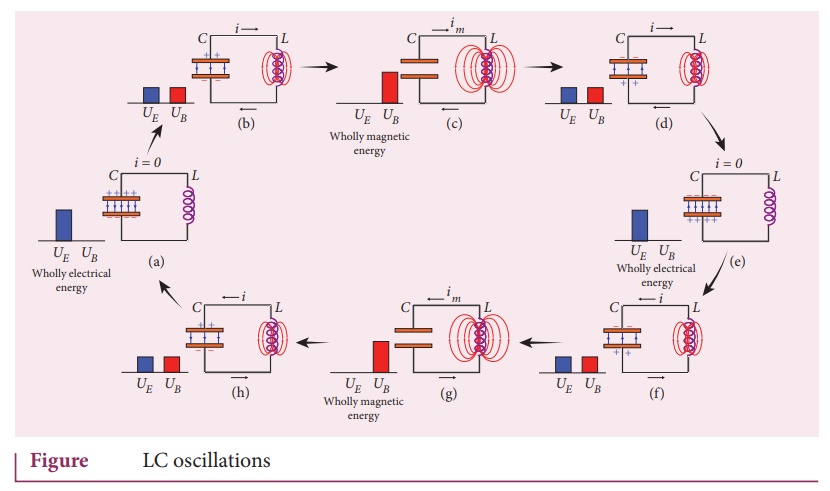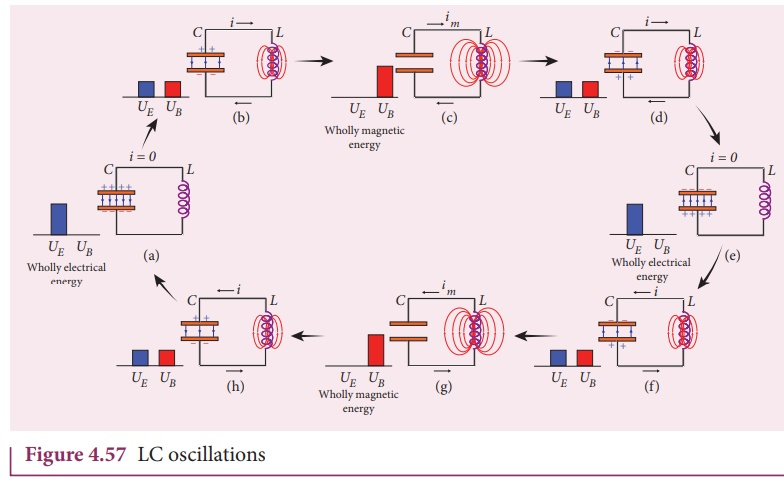Home | | Physics 12th Std | Energy conversion during LC oscillations

# Energy conversion during LC oscillationsWhenever energy is given to a circuit containing a pure inductor of inductance L and a capacitor of capacitance C, the energy oscillates back and forth between the magnetic field of the inductor and the electric field of the capacitor.

Energy conversion during LC oscillations

We have learnt that energy can be stored in both inductors and capacitors. In inductors, the energy is stored in the form of magnetic field while in capacitors; it is stored as the electric field.

Whenever energy is given to a circuit containing a pure inductor of inductance L and a capacitor of capacitance C, the energy oscillates back and forth between the magnetic field of the inductor and the electric field of the capacitor. Thus the electrical oscillations of definite frequency are generated. These oscillations are called LC oscillations.

## Generation of LC oscillations

Let us assume that the capacitor is fully charged with maximum charge Qm at the initial stage. So that the energy stored in the capacitor is maximum and is given by UE=Q2/2C. As there is no current in the inductor, the energy stored in it is zero i.e., UB = 0. Therefore, the total energy is wholly electrical. This is shown in Figure 4.57(a).

The capacitor now begins to discharge through the inductor that establishes current i in clockwise direction. This current produces a magnetic field around the inductor and the energy stored in the inductor is given by UB = Li2 /2 . As the charge in the capacitor decreases, the energy stored in it also decreases and is given by UE = q22. Thus there is a transfer of some part of energy from the capacitor to the inductor.At that instant, the total energy is the sum of electrical and magnetic energies (Figure 4.57(b)).

When the charges in the capacitor are exhausted, its energy becomes zero i.e., UE = 0 . The energy is fully transferred to the magnetic field of the inductor and its energy is maximum. This maximum energy is given by UB=LI m 2/2 Where Im is the maximum  current flowing in the circuit. The total energy is wholly magnetic (Figure 4.57(c)).

Even though the charge in the capacitor is zero, the current will continue to flow in the same direction because the inductor will not allow it to stop immediately. The current is made to flow with decreasing magnitude by the collapsing magnetic field of the inductor. As a result of this, the capacitor begins to charge in the opposite direction. A part of the energy is transferred from the inductor back to the capacitor. The total energy is the sum of the electrical and magnetic energies (Figure 4.57(d)).

When the current in the circuit reduces to zero, the capacitor becomes fully charged in the opposite direction. The energy stored in the capacitor becomes maximum. Since the current is zero, the energy stored in the inductor is zero. The total energy is wholly electrical (Figure 4.57(e)).

The state of the circuit is similar to the initial state but the difference is that the capacitor is charged in opposite direction. The capacitor then starts to discharge through the inductor with anti-clockwise current. The total energy is the sum of the electrical and magnetic energies (Figure 4.57(f)).

As already explained, the processes are repeated in opposite direction (Figure 4.57(g) and (h)). Finally, the circuit returns to the initial state (Figure 4.57(a)). Thus, when the circuit goes through these stages, an alternating current flows in the circuit. As this process is repeated again and again, the electrical oscillations of definite frequency are generated. These are known as LC oscillations.

In the ideal LC circuit, there is no loss of energy. Therefore, the oscillations will continue indefinitely. Such oscillations are called undamped oscillations.

Study Material, Lecturing Notes, Assignment, Reference, Wiki description explanation, brief detail
12th Physics : Electromagnetic Induction and Alternating Current : Energy conversion during LC oscillations |